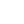# Life 4 J

``````    solveRPN' :: (Num a, Read a) => String -> a
solveRPN' = head . foldl foldingFunction [] . words
where   foldingFunction (x:y:ys) "*" = (x * y):ys
foldingFunction (x:y:ys) "+" = (x + y):ys
foldingFunction (x:y:ys) "-" = (y - x):ys
foldingFunction xs numberString = read numberString:xs

solveRPN' "10 4.0 3 + 2 * -"
By default, the ghc will deduce this function as `(Num Int, Read Int)` . The error is casued by the Prelude.read function which cannot parse a "Floating number string" into Int. However, we can explicity specific the type of expression is Float. Integer string can be parsed into Floating number but Floating number cannot be parsed into Int.
``````    read "4.0" :: Int